## GATE 2017 [Set-1]

 Question 1

The statement (¬p) ⇒ (¬q) is logically equivalent to which of the statements below?

I. p ⇒ q
II. q ⇒ p
III. (¬q) ∨ p
IV. (¬p) ∨ q
 A I only B I and IV only C II only D II and III only
Engineering-Mathematics       Propositional-Logic
Question 1 Explanation:
Method 1:
Construct Truth tables:
~p ⇒ ~q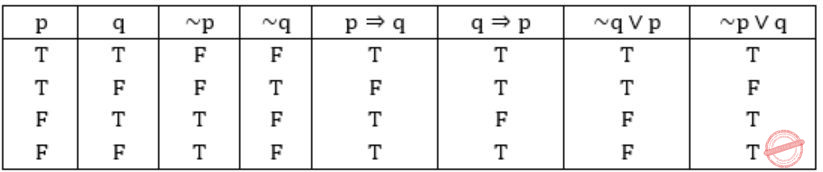II, III are equivalent to (~p) ⇒ (~q)
Method 2:
(I) p⇒q ≡ ~p∨q
(II) q⇒p ≡ ~q∨p
(III) (~q) ∨ p ≡ ~q∨p
(IV) (~p) ∨ p ≡ ~p∨q
Also, from question:
(~p) ⇒ (~q)
≡ p∨~q
So, (II) & (III) are equivalent to the statement given in question.
 Question 2

Consider the first-order logic sentence F: ∀x(∃yR(x,y)). Assuming non-empty logical domains, which of the sentences below are implied by F?

I. ∃y(∃xR(x,y))
II. ∃y(∀xR(x,y))
III. ∀y(∃xR(x,y))
IV. ¬∃x(∀y¬R(x,y))
 A IV only B I and IV only C II only D II and III only
Engineering-Mathematics       Prepositional-Logic
Question 2 Explanation:
Lets convert the given first order logic sentence into some english sentence.
F: ∀x(∃yR(x,y)) (given)
: For all girls there exist a boyfriend
(x for girls and y for boys)
I: ∃y(∃xR(x,y))
: There exist some boys who have girlfriends.
(Subset of statement F, so True)
II: ∃y(∀xR(x,y))
: There exists some boys for which all the girls are girlfriend. (False)
III: ∀y(∃xR(x,y))
: For all boys exists a girlfriend. (False)
IV: ~∃x(∀y~R(x,y))
= ∀x(~∀y~R(x,y))
= ∀x(∃yR(x,y)) (∵ ~∀y=∃y, ~∃x=∀x)
(True)
 Question 3

Let c1, cn be scalars not all zero. Such that the following expression holds:where ai is column vectors in Rn. Consider the set of linear equations.

Ax = B.

where A = [a1.......an] andThen, Set of equations has

 A a unique solution at x = Jn where Jn denotes a n-dimensional vector of all 1 B no solution C infinitely many solutions D finitely many solutions
Engineering-Mathematics       Linear-Algebra
Question 3 Explanation:
ai is a column vector
AX = BAs given thatand c1&cn ≠ 0
means c0a0 + c1a1 + ...cnan = 0, represents that a0, a1... an are linearly dependent.
So rank of 'A' = 0, (so if ‘B’ rank is = 0 infinite solution, ‘B’ rank>0 no solution) ⇾(1)
Another condition given here is, 'Σai = b',
so for c1c2...cn = {1,1,...1} set, it is having value 'b',
so there exists a solution if AX = b →(2)
From (1)&(2), we can conclude that AX = B has infinitely many solutions.
 Question 4

Consider the following functions from positives integers to real numbersThe CORRECT arrangement of the above functions in increasing order of asymptotic complexity is:

 ABC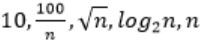DAlgorithms       Asymptotic-Complexity
Question 4 Explanation:
In this problem, they are expecting to find us “increasing order of asymptotic complexity”.
Step-1: Take n=2048 or 211 (Always take n is very big number)
Step-2: Divide functions into 2 ways
1. Polynomial functions
2. Exponential functions
Step-3: The above functions are belongs to polynomial. So, simply substitute the value of n,
First compare with constant values.
→ 100 / 2048 = 0.048828125
→ 10 > 100/ 2048
→ log2 2048 =11
→ √n = 45.25483399593904156165403917471
→ n = 2048
So, Option B is correct
 Question 5

Consider the following tableMatch the algorithm to design paradigms they are based on:

 A (P)↔(ii), Q↔(iii), (R)↔(i) B (P)↔(iii), Q↔(i), (R)↔(ii) C (P)↔(ii), Q↔(i), (R)↔(iii) D (P)↔(i), Q↔(ii), (R)↔(iii)
Algorithms
Question 5 Explanation:
(P) Kruskal’s and Prim’s algorithms for finding Minimum Spanning Tree(MST). To find MST we are using greedy technique.
(Q) QuickSort is a Divide and Conquer algorithm.
(R) Floyd Warshall Algorithm is for solving the All Pairs Shortest Path problem using Dynamic Programming.
Some important points regarding Greedy Vs Dynamic Programming
Greedy: →
It always gives polynomial time complexity
→ It is not an optimal
→ It always selects only either minimum or maximum among all possibilities
→ Ex: Dijkstra’s algorithm for SSSP, Optimal Merge Pattern, Huffman coding, Fractional knapsack problem, etc..,
Dynamic Programming:
→ It gives either polynomial or exponential time complexity.
→ It gives always an optimal result.
→ It checks all possibilities of a problem.
→ Ex: Longest Common sequence, Matrix chain Multiplication, Travelling sales Problem, etc.
 Question 6

Let T be a binary search tree with 15 nodes. The minimum and maximum possible heights of T are:

Note: The height of a tree with a single node is 0.
 A 4 and 15 respectively B 3 and 14 respectively C 4 and 14 respectively D 3 and 15 respectively
Engineering-Mathematics       Binary-Trees
Question 6 Explanation:
T be a Binary Search Tree with 15 nodes.
The height of a tree with single node is 0.
Minimum possible height is when it is a complete binary tree.Maximum possible height is when it is a skewed tree left/right.So the minimum and maximum possible heights of T are: 3 and 14 respectively.
 Question 7

The n-bit fixed-point representation of an unsigned real number X uses f bits for the fraction part. Let i = n-f. The range of decimal values for X in this representation is

 A 2-f to 2i B 2-f to (2i - 2-f) C 0 to 2i D 0 to (2i - 2-f )
Digital-Logic-Design       Number-Systems
Question 7 Explanation:
Size of the fixed point number → n-bits
Number of bits in fraction part → f-bits
Number of bits in integer part → (n – f) bits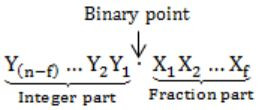Minimum value:
000…0.000…0 = 0
Maximum value:= (2 n-f - 1) + (1 - 2 -f
= (2n-f - 2 -f)
= (2i - 2 -f )
 Question 8

Consider the C code fragment given below.

```typedef struct node   {
int data;
node* next;
}   node;

void join(node* m, node* n)   {
node*  p = n;
while(p→next  !=NULL)    {
p = p→next;
}
p→next=m;
}
```

Assuming that m and n point to valid NULL-terminated linked lists, invocation of join will

 A append list m to the end of list n for all inputs. B either cause a null pointer dereference or append list m to the end of list n. C cause a null pointer dereference for all inputs. D append list n to the end of list m for all inputs.
Question 8 Explanation:
As specified in the question m & n are valid lists, but there is no specific condition/ statement tells that lists are empty or not.
So have to consider both the cases.
⇾ 1. Lists are not null, invocation of JOIN will append list m to the end of list n.
m = 1, 2, 3
n = 4, 5, 6
After join, it becomes 4, 5, 6, 1, 2, 3, NULL.
⇾ 2. If lists are null, if the list n is empty, and itself NULL, then joining & referencing would obviously create a null pointer issue.
Hence, it may either cause a NULL pointer dereference or appends the list m to the end of list n.
 Question 9

When two 8-bit numbers A7...A0 and B7...B0 in 2’s complement representation (with A0 and B0 as the least significant bits) are added using a ripple-carry adder, the sum bits obtained are S7...S0 and the carry bits are C7...C0. An overflow is said to have occurred if

 A the carry bit C7 is 1 B all the carry bits (C7,…,C0) are 1 CDQuestion 9 Explanation:
⇾ Overflow may occur when numbers of same sign are added
i.e., A7 = B7
⇾ Overflow can be detected by checking carry into the sign bits (Cin) and carry out of the sign bits (Cout).
⇾ Overflow occurs iff A7 = B7 and Cin ≠ Cout
These conditions are equivalent toConsider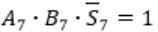Here A7 = B7 = 1 and S7 = 0
This happens only if Cin = 0Carry out Cout=1 when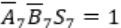Similarly, in case ofCin=1 and Cout will be 0.Question 10

Consider the following context-free grammar over the alphabet Σ = {a, b, c} with S as the start symbol:

S → abScT | abcT
T → bT | b

Which one of the following represents the language generated by the above grammar?

 A {(ab)n (cb)n│n ≥ 1} B {(ab)n cb(m1 ) cb(m2 )…cb(mn )│n,m1,m2,…,mn ≥ 1} C {(ab)n (cbm)n│m,n ≥ 1} D {(ab)n (cbn)m│m,n ≥ 1}
Theory-of-Computation       Contest-Free-Grammar
Question 10 Explanation:
T→ bT | b, this production will generate any number of b’s > 1
S→ abScT | abcT, this production will generate equal number of “ab” and “c” and for every “abc” any number of b’s ( > 1) after “abc”.
For Ex:Hence the language generated by the grammar is
L = {(ab)n cb(m1 ) cb(m2 )…cb(mn )│n,m1,m2,…,mn ≥ 1}
 Question 11

Consider the C struct defined below:

```    struct data   {
int marks ;
int cnumber;
};
struct data student;
```

The base address of student is available in register R1. The field student.grade can be accessed efficiently using

 A Post-increment addressing mode, (R1)+ B Pre-decrement addressing mode, -(R1) C Register direct addressing mode, R1 D Index addressing mode, X(R1), where X is an offset represented in 2’s complement 16-bit representation
Question 11 Explanation:
sruct data
{
int marks;
int cnumber;
}; struct data student
Base Address of student is available in R1.
It is clearly mentioned X is the offset address to be summed with Base Address of R1.Hence Index Addressing mode X(R1), where X is an offset represented in 2’s complement 16-bit representation.
⇾ Relative, Base Indexed & all subtypes of Indirect addressing modes are used with Arrays.
 Question 12

Consider the following intermediate program in three address code

```           p = a - b
q = p * c
p = u * v
q = p + q
```

Which of the following corresponds to a static single assignment form of the above code?

 ABCDCompiler-Design       Static-single-assignment
Question 12 Explanation:
Static Single Assignment is used for intermediate code in compiler design.
In Static Single Assignment form (SSA) each assignment to a variable should be specified with distinct names.
We use subscripts to distinguish each definition of variables.
In the given code segment, there are two assignments of the variable p
p = a-b
p = u*v
and two assignments of the variable q
q = p*c
q = p+q
So we use two variables p1, p2 for specifying distinct assignments of p and q1, q2 for each assignment of q.
Static Single Assignment form(SSA) of the given code segment is:
p1 = a-b
q1 = p1 * c
p2 = u * v
q2 = p2 + q1
Note:
As per options, given in GATE 2017 answer is B.
p3 = a - b
q4 = p3 * c
p4 = u * v
q5 = p4 + q4
 Question 13

Consider the following C code:

```             #include <stdio.h>
int *assignval(int *x, int val)   {
*x = val;
return x;
}

void main ( )  {
int  *x = malloc(sizeof(int));
if(NULL == x)  return;
x = assignval(x, 0);
if(x)  {
x = (int *)malloc(size of(int));
if(NULL == x)  return;
x = assignval(x, 10);
}
printf("%dn",  *x);
free(x);
}
```

The code suffers from which one of the following problems:

 A compiler error as the return of malloc is not typecast approximately B compiler error because the comparison should be made as x==NULL and not as shown C compiles successfully but execution may result in dangling pointer D compiles successfully but execution may result in memory leak
Programming       Programming
Question 13 Explanation:
Option A:
In C++, we need to perform type casting, but in C Implicit type casting is done automatically, so there is no compile time error, it prints10 as output.
Option B:
NULL means address 0, if (a == 0) or (0 == a) no problem, though we can neglect this, as it prints 10.
Option C:
x points to a valid memory location. Dangling Pointer means if it points to a memory location which is freed/ deleted.
int*ptr = (int*)malloc(sizeof(int));
free(ptr); //ptr becomes a dangling pointer
ptr = NULL; //Removing Dangling pointers condition
Option D:
x is assigned to some memory location
int*x = malloc(sizeof(int));
→ (int*)malloc(sizeof(int)) again assigns some other location to x, previous memory location is lost because no new reference to that location, resulting in Memory Leak.
Hence, Option D.
 Question 14

Consider a TCP client and a TCP server running on two different machines. After completing data transfer, the TCP client calls close to terminate the connection and a FIN segment is sent to the TCP server. Server-side TCP responds by sending an ACK, which is received by the client-side TCP. As per the TCP connection state diagram (RFC 793), in which state does the client-side TCP connection wait for the FIN from the server-side TCP?

 A LAST-ACK B TIME-WAIT C FIN-WAIT-1 D FIN-WAIT-2
Computer-Networks       TCP-Transition-Diagram
Question 14 Explanation:
Client has sent FIN segment to the server and moves to FIN-WAIT-1,
i.e. waiting for the ACK for own FIN segment.
There are two possibilities here:
I. If Client receives ACK for its FIN then client will move to FIN-WAIT-2 and will wait for matching FIN from server side.
After receiving the FIN from server, client will send ACK and move to TIME-WAIT state.
II. Client has sent FIN segment but didn’t get ACK till the time.
Client will acknowledge this FIN and move to CLOSE state.
Here Client will wait for the ACK for its own FIN.
After receiving ACK, client will move to TIME-WAIT state.
Here we encounter First Case.
So, the solution is (D).
Refer this TCP state transition diagram:Question 15

A sender S sends a message m to receiver R, which is digitally signed by S with its private key. In this scenario, one or more of the following security violations can take place.

(I) S can launch a birthday attack to replace m with a fraudulent message.
(II) A third party attacker can launch a birthday attack to replace m with a fraudulent message.
(III) R can launch a birthday attack to replace m with a fraudulent message.

Which of the following are possible security violations?

 A (I) and (II) only B (I) only C (II) only D (II) and (III) only
Computer-Networks       Security
Question 15 Explanation:
Birthday attack Problem is when sender replaces original message with fraud message having same message digest as the original message, along with the digital signature of the original message.
(I) Can the sender replace the message with a fraudulent message?
Yes, definitely because the sender will encrypt the message with its private key.
It can encrypt another message also with its private key.
(II) Can the third party send a fraudulent message?
No, because the third party doesn't know about the private key of the sender.
(III) Can receiver send the fraudulent message?
No, the receiver also doesn't know about the Private key of the sender.
So receiver also cannot send the fraudulent message.
 Question 16

The following functional dependencies hold true for the relational schema {V, W, X, Y, Z} :

V → W
VW → X
Y → VX
Y → Z

Which of the following is irreducible equivalent for this set of functional dependencies?

 A V→WV→XY→VY→Z B V→WW→XY→VY→Z C V→WV→XY→VY→XY→Z D V→WW→XY→VY→XY→Z
Database-Management-System       Functional-Dependency
Question 16 Explanation:
Step 1:
V → W, VW → X, Y → V, Y → X, Y→ Z
Step 2:
V → W, VW → X, Y → V, Y → X, Y→ Z
(V)+ = V ×
(VW)+ = VW ×
(Y)+ = YXZ
(Y)+ = YVW ×
(Y)+ = YVWX
Without Y → X, the closure of Y is deriving ‘X’ from the remaining attributes.
So, we can remove Y → X as its redundant.
Step 3:
V → W, VW → X, Y → V, Y → Z
(V)+ = VW, the closure of V is deriving W from the remaining FD’s.
So, W is redundant. We can remove it.
So, the final canonical form is
V→W, V→X, Y→V, Y→Z
⇾ So, option (A) is correct.
 Question 17

Consider the following grammar:What is FOLLOW(Q)?

 A {R} B {w} C {w, y} D {w, \$}
Compiler-Design       First-and-Follow
Question 17 Explanation:
In the production: P → xQRS,
FIRST (R) = {w, ϵ} >br> Since FIRST (R) = {ϵ}, so FOLLOW (Q) → {w} ∪ FIRST(S)
FIRST(S) = {y}
So, FOLLOW (Q) = {w, y}
 Question 18

 A global variables but not heap. B heap but not global variables. C neither global variables nor heap. D both heap and global variables.
Question 18 Explanation:
Thread share all other resources of process except local data like – register, stack.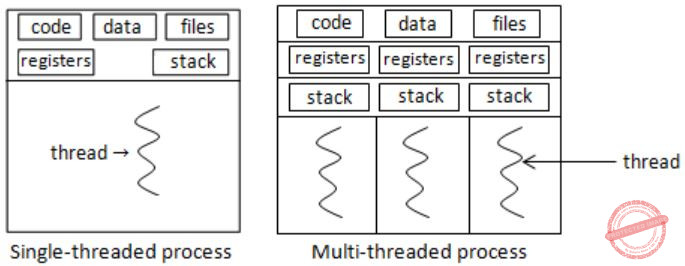Question 19

Let X be a Gaussian random variable with mean 0 and variance σ2. Let Y = max(X, 0) where max(a,b) is the maximum of a and b. The median of Y is __________.

 A 0 B 1 C 2 D 3
Engineering-Mathematics       Probability
Question 19 Explanation:
Given that, Y = max(X,0),which means, Y is 0 for negative values of X and Y is X for positive values of X.
Median is a point, where the probability of getting less than median is 1/2 and probability of getting greater than median is 1/2.
From the given details, we can simply conclude that, median is 0. (0 lies exactly between positive and negative values)Question 20

Let T be a tree with 10 vertices. The sum of the degrees of all the vertices in T is _________.

 A 18 B 19 C 20 D 21
Data-Structures       Trees
Question 20 Explanation:
Sum of degrees of all vertices is double the number of edges.
A tree, with 10 vertices, consists n - 1, i.e. 10 - 1 = 9 edges.
Sum of degrees of all vertices = 2(#edges)
= 2(9)
= 18
 Question 21

Consider the Karnaugh map given below, where X represents “don’t care” and blank represents 0.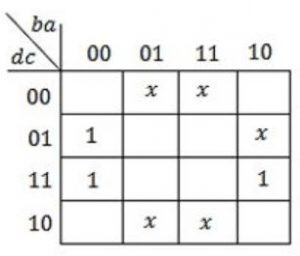Assume for all inputs, the respective complements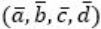are also available. The above logic is implemented using 2-input NOR gates only. The minimum number of gates required is _________.

 A 1 B 2 C 3 D 4
Digital-Logic-Design       K-Map
Question 21 Explanation:
Given K-Map represents the function f(a, b, c, d) = a'c = a'(c')' = (a + c')'
As all variables and their complements are available we can implement the function with only one NOR Gate.
 Question 22

Consider the language L given by the regular expression (a+b)*b(a+b) over the alphabet {a,b}. The smallest number of states needed in deterministic finite-state automation (DFA) accepting L is _________.

 A 4 B 5 C 6 D 7
Theory-of-Computation       Finite-Automata
Question 22 Explanation:
The NFA for regular expression: (a+b)*b(a+b)After converting the NFA into DFA:After converting the NFA into DFA:Question 23

Consider a database that has the relation schema EMP (EmpId, EmpName, and DeptName). An instance of the schema EMP and a SQL query on it are given below.The output of executing the SQL query is ___________.

 A 2.6 B 2.7 C 2.8 D 2.9
Database-Management-System       SQL
Question 23 Explanation:
The given query is⇾ We start evaluating from the inner query.
The inner query forms DeptName wise groups and counts the DeptName wise EmpIds.
⇾ In inner query DeptName, Count(EmpId) is the alias name DeptName, Num.
So, the output of the inner query is,The outer query will find the
Avg(Num) = (4+3+3+2+1)/5 = 2.6
 Question 24

Consider the following CPU processes with arrival times (in milliseconds) and length of CPU bursts (in milliseconds) as given below: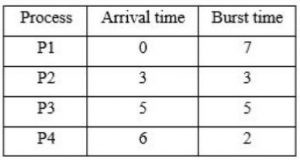If the pre-emptive shortest remaining time first scheduling algorithm is used to schedule the processes, then the average waiting time across all processes is __________ milliseconds.

 A 3 B 4 C 5 D 6
Operating-Systems       Process-Scheduling
Question 24 Explanation:Question 25

Consider a two-level cache hierarchy with L1 and L2 caches. An application incurs 1.4 memory accesses per instruction on average. For this application, the miss rate of L1 cache is 0.1; the L2 cache experiences, on average, 7 misses per 1000 instructions. The miss rate of L2 expressed correct to two decimal places is __________.

 A 0.05 B 0.06 C 0.07 D 0.08
Computer-Organization       Cache
Question 25 Explanation:
It is given that average references per instruction = 1.4
For 1000 instructions total number of memory references = 1000 * 1.4 = 1400
These 1400 memory references are first accessed in the L1.
Since the miss rate of L1 is 0.1, for 1400 L1 references the number of misses = 0.1 * 1400 = 140
We know when there is a miss in L1 we next access the L2 cache.
So number of memory references to L2 = 140
It is given that there are 7 misses in L2 cache. Out of 140 memory references to L2 cache there are 7 misses.
Hence the miss rate in L2 cache = 7/140 = 0.05
 Question 26

Let G = (V, E) be any connected undirected edge-weighted graph. The weights of the edges in E are positive and distinct. Consider the following statements:

(I) Minimum Spanning Tree of G is always unique.
(II) Shortest path between any two vertices of G is always unique.

Which of the above statements is/are necessarily true?

 A (I) only B (II) only C both (I) and (II) D neither (I) nor (II)
Algorithms       Minimum-Spanning-Tree
Question 26 Explanation:
If the graph has all positive and distinct (unique values no duplicates) then Statement-I definitely correct because if we are using either prim’s or kruskal’s algorithm it gives the unique spanning tree.
Let us take an exampleStep 1:
Using kruskal’s algorithm, arrange each weights in ascending order.
17, 18, 20, 21, 22, 23, 24, 25, 26, 27, 28, 29, 30
Step 2:Step 3:
17+18+20+21+22+23+26 = 147
Step 4:
Here, all the elements are distinct. So the possible MCST is 1.
Statement-II: May or may not happen, please take an example graph and try to solve it. This is not correct always.
So, we have to pick most appropriate answer.
 Question 27

A multithreaded program P executes with x number of threads and used y number of locks for ensuring mutual exclusion while operating on shared memory locations. All locks in the program are non-reentrant, i.e., if a thread holds a lock l, then it cannot re-acquire lock l without releasing it. If a thread is unable to acquire a lock, it blocks until the lock becomes available. The minimum value of x and the minimum value of y together for which execution of P can result in a deadlock are:

 A x = 1, y = 2 B x = 2, y = 1 C x = 2, y = 2 D x = 1, y = 1
Operating-Systems       Process-Synchronization
Question 27 Explanation:
First you have to know multithreading, mutual exclusion and reentrant mutex. The reentrant mutex (recursive mutex, recursive lock) is particular type of mutual exclusion (mutex) device that may be locked multiple times by the same process/thread, without causing a deadlock.
Here non re-entrant process can’t own same lock multiple times, so if process tries to acquire already owned lock, will get blocked, and deadlock will happen.
From the above options x=1 (a single thread) and y=1 (a single lock) deadlock is possible when we consider given situations in question.
 Question 28
The value ofA is 0 B is -1 C is 1 D does not exist
Engineering-Mathematics       Calculus
Question 28 Explanation:If "x=1" is substituted we get 0/0 form, so apply L-Hospital ruleSubstitute x=1
⇒ (7(1)6-10(1)4)/(3(1)2-6(1)) = (7-10)/(3-6) = (-3)/(-3) = 1Question 29

Let p, q and r be prepositions and the expression (p → q) → r be a contradiction. Then, the expression (r → p) → q is

 A a tautology B a contradiction C always TRUE when p is FALSE D always TRUE when q is TRUE
Engineering-Mathematics       Prepositional-Logic
Question 29 Explanation:
Given that (p→q)→r is a contradiction.
So r = F and (p→q) = T.
We have to evaluate the expression
(r→p)→q
Since r = F, (r→p) = T (As F→p, is always true)
The final expression is T→q and this is true when q is true, hence option D.
 Question 30

Let u and v be two vectors in R2 whose Euclidean norms satisfy ||u||=2||v||. What is the value of α such that w = u + αv bisects the angle between u and v?

 A 2 B 1/2 C 1 D -1/2
Engineering-Mathematics       Linear-Algebra
Question 30 Explanation:Let u, v be vectors in R2, increasing at a point, with an angle θ.
A vector bisecting the angle should split θ into θ/2, θ/2
Means ‘w’ should have the same angle with u, v and it should be half of the angle between u and v.
Assume that the angle between u, v be 2θ (thus angle between u,w = θ and v,w = θ)
Cosθ = (u∙w)/(∥u∥ ∥w∥) ⇾(1)
Cosθ = (v∙w)/(∥v∥ ∥w∥) ⇾(2)
(1)/(2) ⇒ 1/1 = ((u∙w)/(∥u∥ ∥w∥))/((v∙w)/(∥v∥ ∥w∥)) ⇒ 1 = ((u∙w)/(∥u∥))/((v∙w)/(∥v∥))
⇒ (u∙w)/(v∙w) = (∥u∥)/(∥v∥) which is given that ∥u∥ = 2 ∥v∥
⇒ (u∙w)/(v∙w) = (2∥v∥)/(∥v∥) = 2 ⇾(3)
Given ∥u∥ = 2∥v∥
u∙v = ∥u∥ ∥v∥Cosθ
=2∙∥v∥2 Cosθ
w = u+αv
(u∙w)/(v∙w) = 2
(u∙(u+αv))/(v∙(u+αv)) = 2
(u∙u+α∙u∙v)/(u∙v+α∙v∙v) = 2a∙a = ∥a∥2
4∥v∥2+α∙2∙∥v∥2 Cosθ = 2(2∥v∥2 Cosθ+α∙∥v∥2)
4+2αCosθ = 2(2Cosθ+α)
4+2αCosθ = 4Cosθ+2α ⇒ Cosθ(u-v)+2α-4 = 0
4-2α = Cosθ(4-2α)
(4-2α)(Cosθ-1) = 0
4-2α = 0Question 31

Let A be m×n real valued square symmetric matrix of rank 2 with expression given below.Consider the following statements

(i) One eigenvalue must be in [-5, 5].
(ii) The eigenvalue with the largest magnitude must be strictly greater than 5.

Which of the above statements about engenvalues of A is/are necessarily CORRECT?

 A Both (I) and (II) B (I) only C (II) only D Neither (I) nor (II)
Engineering-Mathematics       Linear-Algebra
Question 31 Explanation:
Letbe a real valued, rank = 2 matrix.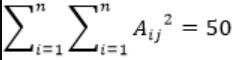a2+b2+c2+d2 = 50
Square values are of order 0, 1, 4, 9, 16, 25, 36, …
So consider (0, 0, 5, 5) then Sum of this square = 0+0+25+25=50
To get rank 2, the 2×2 matrix can beThe eigen values are,
|A-λI| = 0 (The characteristic equation)-λ(-λ)-25 = 0
λ2-25 = 0So, the eigen values are within [-5, 5], Statement I is correct.
The eigen values with largest magnitude must be strictly greater than 5: False.
So, only Statement I is correct.
 Question 32

A computer network uses polynomials over GF(2) for error checking with 8 bits as information bits and uses x3 + x + 1 as the generator polynomial to generate the check bits. In this network, the message 01011011 is transmitted as

 A 01011011010 B 01011011011 C 01011011101 D 01011011100
Computer-Networks       CRC
Question 32 Explanation:
Given CRC generator polynomial = x3+x+1
= 1∙x3+0∙x2+1∙x1+1∙x0
= 1011
Message = 01011011So, the message 01011011 is transmitted asQuestion 33

Consider a combination of T and D flip-flops connected as shown below. The output of the D flip-flop is connected to the input of the T flip-flop and the output of the T flip-flop is connected to the input of the D flip-flop.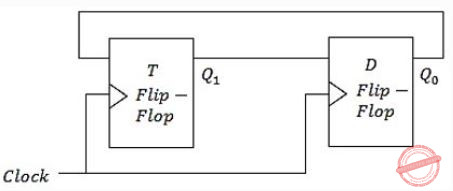Initially, both Q0 and Q1 are set to 1 (before the 1st clock cycle). The outputs

 A Q1Q0 after the 3rd cycle are 11 and after the 4th cycle are 00 respectively B Q1Q0 after the 3rd cycle are 11 and after the 4th cycle are 01 respectively C Q1Q0 after the 3rd cycle are 00 and after the 4th cycle are 11 respectively D Q1Q0 after the 3rd cycle are 01 and after the 4th cycle are 01 respectively
Digital-Logic-Design       Sequential-Circuits
Question 33 Explanation: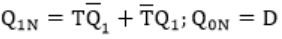Question 34

If G is a grammar with productions

S → SaS | aSb | bSa | SS | ϵ

where S is the start variable, then which one of the following strings is not generated by G?

 A abab B aaab C abbaa D babba
Theory-of-Computation       Membership-Function
Question 34 Explanation:
The strings “abab”, “aaab” and “abbaa” can be generated by the given grammar.But the string “babba” can’t be generated by the given grammar.
The reason behind this is, we can generate any number of a’s with production S→ SaS, but for one “b” we have to generate one “a”, as the production which is generating “b” is also generating “a” together (S→ aSb and S→ bSa).
So in string “babba” the first and last “ba” can be generated by S→ bSa, but we can’t generate a single “b” in middle.
In other words we can say that any string in which number of “b’s” is more than number of “a’s” can’t be generated by the given grammar.
 Question 35

Consider the following two functions.

```void fun1(int n)   {                 void fun2(int n)   {
if(n == 0)  return;                  if(n == 0)  return;
printf("%d", n);                     printf("%d", n);
fun2(n - 2);                         fun1(++n);
printf("%d", n);                     printf("%d", n);
}                                    }
```

The output printed when fun1(5) is called is

 A 53423122233445 B 53423120112233 C 53423122132435 D 53423120213243
Programming       Programming
Question 35 Explanation: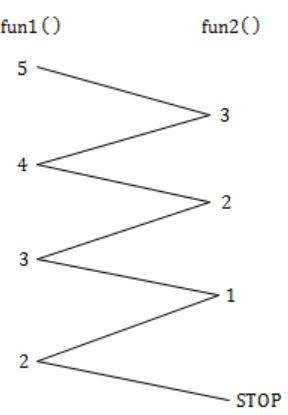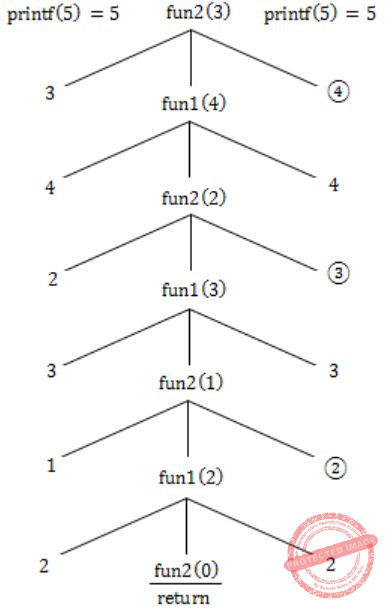In fun2, we increment (pre) the value of n, but in fun1, we are not modifying the value.
Hence increment in value in recursion (back).
Hence, 5 3 4 2 3 1 2 2 2 3 3 4 4 5.
 Question 36

Consider the C functions foo and bar given below:

```         int foo(int val)   {
int x = 0;
while (val>0)   {
x = x + foo(val--);
}
return val;
}

int bar(int val)   {
int x = 0;
while (val>0)   {
x = x + bar(val-1);
}
return val;
}```

Invocations of foo(3) and bar(3) will result in:

 A Return of 6 and 6 respectively. B Infinite loop and abnormal termination respectively. C Abnormal termination and infinite loop respectively. D Both terminating abnormally.
Programming       Programming
Question 36 Explanation:
while(val>0)
{
x = x + foo(val--);
}
In this case foo(val--) is same as foo(val) & val-- ;
Because the recursive function call is made without changing the passing argument and there is no Base condition which can stop it.
It goes on calling with the same value ‘val’ & the system will run out of memory and hits the segmentation fault or will be terminated abnormally.
The loop will not make any difference here.
while(val>0)
{
x = x + bar(val-1);
}
bar(3) calls bar(2)
bar(2) calls bar(1)
bar(1) calls bar(0) ⇾ Here bar(0) will return 0.
bar(1) calls bar(0)
bar(1) calls bar(0)……..
This will continue.
Here is a problem of infinite loop but not abrupt termination.
Some compilers will forcefully preempt the execution.
 Question 37

Consider the context-free grammars over the alphabet {a, b, c} given below. S and T are non-terminals.

G1: S → aSb|T, T → cT|ϵ
G2: S → bSa|T, T → cT|ϵ

The language L(G1) ∩ L(G2) is

 A Finite B Not finite but regular C Context-Free but not regular D Recursive but not context-free
Theory-of-Computation       Context-Free-Language
Question 37 Explanation:
Strings generated by G1:
{ϵ, c, cc, ccc, … ab, aabb, aaabbb….acb, accb… aacbb, aaccbb, …}
Strings generated by G2:
{ϵ, c, cc, ccc, … ba, bbaa, bbbaaa….bca, bcca… bbcaa, bbccaa, …}
The strings common in L (G1) and L (G2) are:
{ϵ, c, cc, ccc…}
So, L (G1) ∩ L (G2) can be represented by regular expression: c*
Hence the language L (G1) ∩ L (G2) is “Not finite but regular”.
 Question 38

Consider the following languages over the alphabet Σ = {a, b, c}.

Let L1 = {an bn cm│m,n ≥ 0} and L2 = {am bn cn│m,n ≥ 0}

Which of the following are context-free languages?

I. L1 ∪ L2
II. L1 ∩ L2
 A I only B II only C I and II D Neither I nor II
Theory-of-Computation       Context-Free-Language
Question 38 Explanation:
Strings in L1 = {ϵ, c, cc, …., ab, aabb,…., abc, abcc,…, aabbc, aabbcc, aabbccc,..}
Strings in L2 = {ϵ, a, aa, …., bc, bbcc,…., abc, aabc,…, abbcc, aabbcc, aaabbcc,..}
Strings in L1 ∪ L2 ={ϵ, a, aa, .., c, cc,.. ab, bc, …, aabb, bbcc,.., abc, abcc, aabc,…}
Hence (L1 ∪ L2) will have either (number of a’s = equal to number of b’s) OR (number of b’s = number of c’s).
Hence (L1 ∪ L2) is CFL.
Strings in L1 ∩ L2 = {ϵ, abc, aabbcc, aaabbbccc,…}
Hence (L1 ∩ L2) will have (number of a’s = number of b’s = number of c’s)
i.e., (L1 ∩ L2) = {anbncn | n ≥ 0} which is CSL.
 Question 39

Let A and B be finite alphabets and let # be a symbol outside both A and B. Let f be a total function from A* to B*. We say f is computable if there exists a Turing machine M which given an input x in A*, always halts with f(x) on its tape. Let Lf denote the language {x # f(x)│x ∈ A*}. Which of the following statements is true:

 A f is computable if and only if Lf is recursive. B f is computable if and only if Lf is recursively enumerable. C If f is computable then Lf is recursive, but not conversely. D If f is computable then Lf is recursively enumerable, but not conversely.
Theory-of-Computation       Computability-and-Decidability
Question 39 Explanation:
Total function is synonym of function.
Total function means for every element in domain, there must be a mapping in range.
Let us consider A= {a, b} and B = {0,1}
The concept of computing has been intuitively linked with the concept of functions.
A computing machine can only be designed for the functions which are computable.
The basic definition is:
Given a recursive language L and a string w over Σ*, the characteristic function is given byThe function “f” is computable for every value of "w".
However if the language L is not recursive, then the function f may or may not be computable.
Hence, f is computable iff Lf is recursive.
 Question 40

Recall that Belady’s anomaly is that the page-fault rate may increase as the number of allocated frames increases. Now, consider the following statements:

S1: Random page replacement algorithm (where a page chosen at random is replaced) suffers from Belady’s anomaly
S2: LRU page replacement algorithm suffers from Belady’s anomaly

Which of the following is CORRECT?

 A S1 is true, S2 is true B S1 is true, S2 is false C S1 is false, S2 is true D S1 is false, S2 is false
Operating-Systems       Page-Replacement-Algorithm
Question 40 Explanation:
FIFO may suffer from Belady's anomaly not always FIFO suffer from Belady's anomaly.
Page replacement algorithm suffers from Belady's anomaly when it is not a stack algorithm.
S1: Random page replacement algorithm is not a stack algorithm. So, S1 is true.
S2: LRU is a stack algorithm. Therefore, it doesn't suffer from Belady's anomaly. S2 is false.
 Question 41

Consider a database that has the relation schemas EMP(EmpId, EmpName, DeptId), and DEPT(DeptName, DeptId). Note that the DeptId can be permitted to a NULL in the relation EMP. Consider the following queries on the database expressed in tuple relational calculus.

(I) {t│∃u ∈ EMP(t[EmpName] = u[EmpName] ∧ ∀v ∈ DEPT(t[DeptId] ≠ v[DeptId]))}
(II) {t│∃u ∈ EMP(t[EmpName] = u[EmpName] ∧ ∃v ∈ DEPT(t[DeptId] ≠ v[DeptId]))}
(III) {t│∃u ∈ EMP(t[EmpName] = u[EmpName] ∧ ∃v ∈ DEPT(t[DeptId] = v[DeptId]))}

Which of the above queries are safe?

 A (I) and (II) only B (I) and (III) only C (II) and (III) only D (I), (II) and (III)
Database-Management-System       Relational-Algebra
Question 41 Explanation:
An expression is said to be safe, if it yield a finite number of tuples, otherwise unsafe.
(I) Gives EmpNames who do not belong to any Department. So, it is going to be a finite number of tuples as a result.
(II) Gives EmpNames who do not belong to some Department. This is also going to have finite number of tuples.
(III) Gives EmpNames who do not belong to same Department. This one will also give finite number of tuples.
All the expressions I, II and III are giving finite number of tuples. So, all are safe.
 Question 42

In a database system, unique timestamps are assigned to each transaction using Lamport’s logical clock. Let TS(T1) and TS(T2) be the timestamps of transactions T1 and T2 respectively. Besides, T1 holds a lock on the resource R and T2 has requested a conflicting lock on the same resource R. The following algorithm is used to prevent deadlocks in the database system assuming that a killed transaction is restarted with the same timestamp.

```
if TS(T2)<TS(T1) then
T1 is killed
else T2 waits.
```

Assume any transaction that is not killed terminates eventually. Which of the following is TRUE about the database system that uses the above algorithm to prevent deadlocks?

 A The database system is both deadlock-free and starvation-free. B The database system is deadlock-free, but not starvation-free. C The database system is starvation-free, but not deadlock-free. D The database system is neither deadlock-free nor starvation-free.
Database-Management-System       Time-stamp-Ordering
Question 42 Explanation:
In the question, it is given that ‘unique time stamps’ are assigned to the transactions.
So, there will be no equal timestamps.
Lamport’s logical clock:
In this timestamps are assigned in increasing order only.
According to the given algorithm,
TS(T2) < TS(T1)
then T1 is killed
else
T2 will wait
So, in both the cases, it will be deadlock free and there will be no starvation.
 Question 43

Consider the following grammar:

```    stmt    →  if expr then expr else expr; stmt | ȯ
expr    →  term relop term | term
term    →  id | number
id      →  a | b |  c
number  → [0-9]
```

where relop is a relational operator (e.g., <, >, …), ȯ refers to the empty statement, and if, then, else are terminals.

Consider a program P following the above grammar containing ten if terminals. The number of control flow paths in P is ________. For example, the program

```     if e1 then e2 else e3
```

has 2 control flow paths, e1 e2 and e1 e3.

 A 1024 B 1025 C 1026 D 1027
Compiler-Design       Parsers
Question 43 Explanation:
To get 10 'if' we need to use grammar to get,
if then else ; stmt
if then else ; if then else . stmt
:
:
:
(keep doing 10 times to get 10 'if')
We know that every if statement has 2 control flows as given in question. Hence,
We have 2 control flow choices for 1st 'if'
We have 2 control flow choices for 2nd 'if'
:
:
:
We have 2 control flow choices for 10th 'if'
Since all the choices are in one single structure or combination, so total choices are
2 × 2 × 2 × ........ 10 times = 210 = 1024
 Question 44

In a RSA cryptosystem, a participant A uses two prime numbers p = 13 and q = 17 to generate her public and private keys. If the public key of A is 35, then the private key of A is _________.

 A 11 B 12 C 13 D 14
Computer-Networks       RSA-Algorithm
Question 44 Explanation:
Given, p=13, q=17, e=35 (Public key), d=? (Private key)
As per RSA Algorithm; following steps:
Step 1: Find n = p×q = 13×17 = 221
Step 2: Find ∅(n) = (p-1)×(q-1) = 12×16 = 192
Step 3: d×e mod ∅(n) = 1 ⇒ (d = e(-1) mod ∅(n))
or
d×e = 1 mod ∅(n)
⇒ d×35 mod 192 = 1Question 45

The value of parameters for the Stop-and-Wait ARQ protocol are as given below:

```          Bit rate of the transmission channel = 1 Mbps.
Propagation delay from sender to receiver = 0.75 ms.
Time to process a frame = 0.25 ms.
Number of bytes in the information frame = 1980.
Number of bytes in the acknowledge frame = 20.
Number of overhead bytes in the information frame = 20.
```

Assume that there are no transmission errors. Then, the transmission efficiency (expressed in percentage) of the Stop-and-Wait ARQ protocol for the above parameters is _________ (correct to 2 decimal places).

 A 89.33% B 89.34% C 89.35% D 89.36%
Computer-Networks       Stop-and-Wait-ARQ-Protocol
Question 45 Explanation:
Given Data:
B = 1Mbps, L = 1980Bytes, Overhead = 20Bytes
TProc = 0.25ms, LAck = 20Bytes
Tp=0.75ms
Total Data size(L) = (L + overhead) = 1980+20 = 2000Bytes
Efficiency of Stop & Wait ARQ?
Tt = L/B = 2000Bytes/1Mbps = (2000×8bits)/(106 b/s) = 16msec
TAck = LAck/B = (20×8bits)/(106 bits/sec) = 0.16msec
∴ In Stop and Wait ARQ, efficiency
ƞ = Tt/(Tt+TAck+2Tp+TProc) = 16ms/(16+0.16+2×0.75+0.25ms) = 16ms/17.91ms = 0.8933Question 46

Consider a database that has the relation schema CR(StudentName, CourseName). An instance of the schema CR is as given below.The following query is made on the database.

```         T1 ← πCourseName(σStudentName='SA'(CR))
T2 ← CR ÷ T1
```

The number of rows in T2 is ____________.

 A 4 B 5 C 6 D 7
Database-Management-System       Relational-Algebra
Question 46 Explanation:
(1) T1 ← πCourseNameStudentName = 'SA'(CR))
The σStudentName = 'SA'(CR) will produce the following⇾ The result of T1 ← πCourseNameStudentName='SA'(CR)) is(2) T2 ← CR÷T1
⇾ We see that SA is enrolled for CA, CB and CC.
⇾ T2 will give the StudentNames those who have enrolled for all the CA, C, CC courses. So, the following Students are enrolled for the given 3 courses.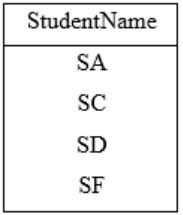⇾ So, the output of T2 will have 4 rows.
 Question 47

The number of integers between 1 and 500 (both inclusive) that are divisible by 3 or 5 or 7 is _________.

 A 271 B 272 C 273 D 274
Engineering-Mathematics       Combinatorics
Question 47 Explanation: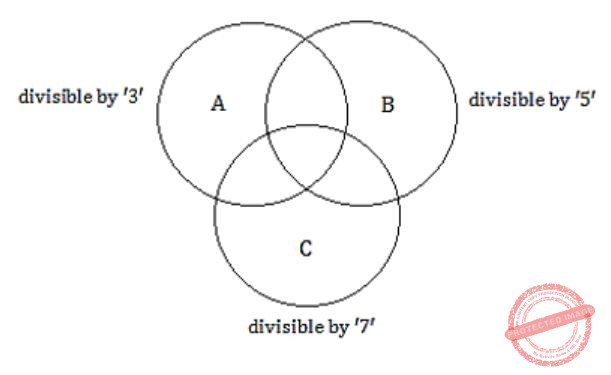Let A = number divisible by 3
B = numbers divisible by 5
C = number divisible by 7
We need to find “The number of integers between 1 and 500 that are divisible by 3 or 5 or 7" i.e., |A∪B∪C|
We know,
|A∪B∪C| = |A|+|B|+C-|A∩B|-|A∩C|-|B∩C|+|A∩B|
|A| = number of integers divisible by 3
[500/3 = 166.6 ≈ 166 = 166]
|B| = 100
[500/5 = 100]
|C| = 71
[500/7 = 71.42]
|A∩B| = number of integers divisible by both 3 and 5 we need to compute with LCM (15)
i.e.,⌊500/15⌋ ≈ 33
|A∩B| = 33
|A∩C| = 500/LCM(3,7) 500/21 = 23.8 ≈ 28
|B∩C| = 500/LCM(5,3) = 500/35 = 14.48 ≈ 14
|A∩B∩C| = 500/LCM(3,5,7) = 500/163 = 4.76 ≈ 4
|A∪B∪C| = |A|+|B|+|C|-|A∩B|-|A∩C|-|B∩C|+|A∩B∩C|
= 166+100+71-33-28-14+4
= 271
 Question 48

Let A be an array of 31 numbers consisting of a sequence of 0’s followed by a sequence of 1’s. The problem is to find the smallest index i such that A[i] is 1 by probing the minimum number of locations in A. The worst case number of probes performed by an optimal algorithm is _________.

 A 5 B 6 C 7 D 8
Algorithms       Searching
Question 48 Explanation:
→ If we apply binary search to find the first occurrence of 1 in the list, it will give us the smallest index i where 1 is stored.
→ As in this array sequence of 0’s is followed by sequence of 1’s, the array is sorted. We can apply binary search directly without sorting it.
So number of probes = ceil(log2 31) = 4.954196310386876
⇒ here we are using ceiling so it becomes 5
 Question 49

Consider a RISC machine where each instruction is exactly 4 bytes long. Conditional and unconditional branch instructions use PC-relative addressing mode with Offset specified in bytes to the target location of the branch instruction. Further the Offset is always with respect to the address of the next instruction in the program sequence. Consider the following instruction sequenceIf the target of the branch instruction is i, then the decimal value of the Offset is ___________.

 A -16 B -17 C -18 D -19
Computer-Organization       Instruction-Cycle
Question 49 Explanation:
Size of each instruction = 4 Bytes
⇾ Assuming the first instruction starts at address zero⇒ Offset = 0 – 16 = (-16) ⇾ Final answer
 Question 50

Instruction execution in a processor is divided into 5 stages, Instruction Fetch (IF), Instruction Decode (ID), Operand Fetch (OF), Execute (EX), and Write Back (WB). These stages take 5, 4, 20, 10 and 3 nanoseconds (ns) respectively. A pipelined implementation of the processor requires buffering between each pair of consecutive stages with a delay of 2 ns. Two pipelined implementations of the processor are contemplated:

(i) a naive pipeline implementation (NP) with 5 stages and
(ii) an efficient pipeline (EP) where the OF stage is divided into stages OF1 and OF2 with execution times of 12 ns and 8 ns respectively.

The speedup (correct to two decimal places) achieved by EP over NP in executing 20 independent instructions with no hazards is _________.

 A 1.51 B 1.52 C 1.53 D 1.54
Computer-Organization       Pipelining
Question 50 Explanation:
Naive Pipeline implementation:
The stage delays are 5, 4, 20, 10 and 3. And buffer delay = 2ns
So clock cycle time = max of stage delays + buffer delay
= max(5, 4, 20, 10,3)+2
= 20+2
= 22ns
Execution time for n-instructions in a pipeline with k-stages = (k+n-1) clock cycles
= (k+n-1)* clock cycle time
In this case execution time for 20 instructions in the pipeline with 5-stages
= (5+20-1)*22ns
= 24*22
= 528ns
Efficient Pipeline implementation:
OF phase is split into two stages OF1, OF2 with execution times of 12ns, 8ns
New stage delays in this case = 5, 4, 12, 8, 10, 3
Buffer delay is the same 2ns.
So clock cycle time = max of stage delays + buffer delay
= max(5, 4, 12, 8, 10,3) + 2
= 12+2
= 14ns
Execution time = (k+n-1) clock cycles
= (k+n-1)* clock cycle time
In this case no. of pipeline stages, k = 6
No. of instructions = 20
Execution time = (6+20-1)*14 = 25*14 = 350ns
Speed up of Efficient pipeline over native pipeline
= Naive pipeline execution time / efficient pipeline execution time
= 528 / 350
≌ 1.51
 Question 51

Consider a 2-way set associative cache with 256 blocks and uses LRU replacement. Initially the cache is empty. Conflict misses are those misses which occur due to contention of multiple blocks for the same cache set. Compulsory misses occur due to first time access to the block. The following sequence of accesses to memory blocks

(0, 128, 256, 128, 0, 128, 256, 128, 1, 129, 257, 129, 1, 129, 257, 129)

is repeated 10 times. The number of conflict misses experienced by the cache is __________.

 A 76 B 79 C 80 D 81
Computer-Organization       Cache
Question 51 Explanation:
When a block is first time accessed and it will not be present in the cache, so it will be a compulsory miss.
If a block is accessed once and then before its second access if there are k-unique block accesses and the cache size is less than k, and in that case if the second access is amiss then it is capacity miss. In this case the cache doesn't have the size to hold all the k-unique blocks that came and so when the initial block came back again it is not in the cache because capacity of the cache is less than the unique block accesses k. Hence it is capacity miss.
If a block is accessed once and then before its second access if there are k-unique block accesses and the cache size is greater than k, and in that case if the second access is a miss then it is conflict miss. In this case the cache can hold all the k-unique blocks that came and even then when the initial block came back again it is not in the cache because it got replaced, then it is conflict miss.
LRU will use the function xmod128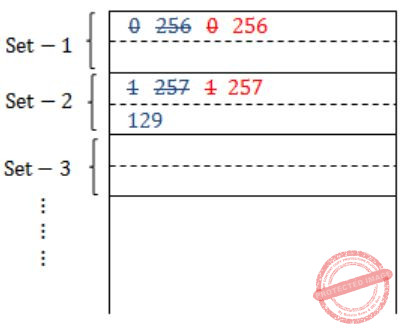Cache size = 256 Bytes
2 way set associative cache
So, no. of cache sets = 256/2 = 128
Blue → Compulsory miss
Red → Conflict miss
At the end of first round we have 4, compulsory misses & 4 conflict misses.
Similarly, if we continue from Round-2 to last round, in every round we will get 8 conflict misses.
Total conflict misses = 4+9(8) = 4+72 = 76 (conflict misses)
 Question 52

Consider the expression (a-1)*(((b+c)/3)+d)). Let X be the minimum number of registers required by an optimal code generation (without any register spill) algorithm for a load/store architecture, in which (i) only load and store instructions can have memory operands and (ii) arithmetic instructions can have only register or immediate operands. The value of X is ___________.

 A 2 B 3 C 4 D 5
Compiler-Design       Register-Allocation
Question 52 Explanation:
(a-1)*(((b+c)/3)+d)Question 53

Consider the following C program.

```#include <stdio.h>
#include <string.h>

void printlength (char*s, char*t)   {
unsigned int c = 0;
int len = ((strlen(s) - strlen(t)) > c) ? strlen(s):strlen(t);
printf("%d\n", len);
}

void main ()  {
char*x = "abc";
char*y = "defgh";
printlength(x,y);
}
```

Recall that strlen is defined in string.h as returning a value of type size_t, which is an unsigned int. The output of the program is _________.

 A 3 B 4 C 5 D 6
Programming       Programming
Question 53 Explanation:
main ( )
{
char*x = "abc";
char*y = "defgh";
printlength(x,y);
}
printlength(char*3, char*t)
{
unsigned int c = 0;
int len = ((strlen(s) - strlen(t))> c) ? strlen(s) : strlen(t);
printf("%d", len);
}
Here strlen(s) - strlen(t) = 3 - 5 = -2
But in C programming, when we do operations with two unsigned integers, result is also unsigned. (strlen returns size_t which is unsigned in most of the systems).
So this result '-2' is treated as unsigned and its value is INT_MAX-2.
Now the comparison is in between large number & another unsigned number c, which is 0.
So the comparison returns TRUE here.
Hence (strlen(s) - strlen(t))>0 will return TRUE, on executing, the conditional operator will return strlen(s)
⇒ strlen(abc) = 3
 Question 54

A cache memory unit with capacity of N words and block size of B words is to be designed. If it is designed as a direct mapped cache, the length of the TAG field is 10 bits. If the cache unit is now designed as a 16-way set-associative cache, the length of the TAG field is ___________ bits.

 A 14 B 15 C 16 D 17
Computer-Organization       Cache
Question 54 Explanation:
When it is directed mapped cache, the physical address can be divided as
(Tag bits + bits for block number + bits for block offset)
With block size being B words no. of bits for block offset = log (B)
Because the cache capacity is N words and each block is B words, number of blocks in cache = N / B
No. of bits for block number = log (N/B)
So, the physical address in direct mapping case
= 10 + log (N/B) + log (B)
= 10 + log (N) – log B + log B
= 10 + log (N)
If the same cache unit is designed as 16-way set associative, then the physical address becomes
(Tag bits + bits for set no. + Bits for block offset)
There are N/B blocks in the cache and in 16-way set associative cache each set contains 16 blocks.
So no. of sets = (N/B) / 16 = N / (16*B)
Then bits for set no = log (N/16B)
Bits for block offset remain the same in this case also. That is log (B).
So physical address in the set associative case
= tag bits + log (N/16*B) + log B
= tag bits + log (N) – log (16*B) + log B
= tag bits + log (N) – log 16 – log B + log B
= tag bits + log N – 4
The physical address is the same in both the cases.
So, 10 + log N = tag bits + log N – 4
Tag bits = 14
So, no. of tag bits in the case 16-way set associative mapping for the same cache = 14.
 Question 55

The output of executing the following C program is __________.

```           #include<stdio.h>
int total (int v)   {
static int count=0;
while(v)   {
count += v&1;
v ≫= 1;
}
return count;
}

void main()    {
static int x = 0;
int i = 5;
for(; 1> 0; i--)    {
x = x + total(i);
}
printf("%d\n", x);
}
```
 A 23 B 24 C 25 D 26
Programming       Programming
Question 55 Explanation:
Arithmetic operators have least priority in this case, as count+=v & 1, we first compute v& 1 and then adds to count variable.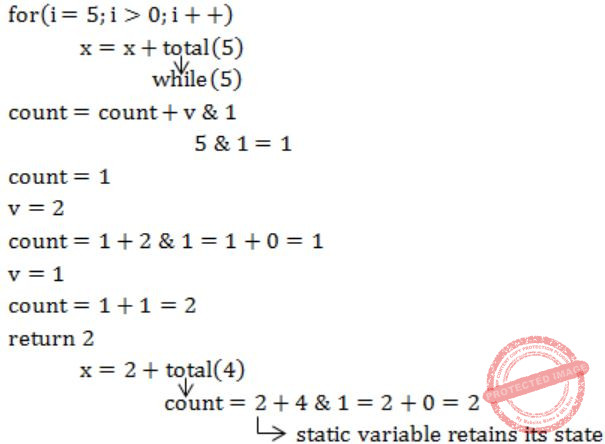Question 56

After RajendraChola returned from his voyage to Indonesia, he _________ to visit the temple in Thanjavur.

 A was wishing B is wishing C wished D had wished
Aptitude       Verbal
Question 56 Explanation:
When the main clause is in the past or past perfect tense, the subordinate clause must be in the past or past perfect tense.
After Rajendrachola returned from his voyage to Indonesia, he wished to visit the temple in Tanjavur.
 Question 57

Research in the workplace reveals that people work for many reason __________.

 A money beside B beside money C money besides D besides money
Aptitude       Verbal
Question 57 Explanation:
Besides is an adverb (or) preposition and it is a preposition it means in addition to apart from as an adverb it means furthermore (or) as well.
Here we are using besides preposition "in addition to".
 Question 58

Rahul, Murali, Srinivas and Arul are seated around a square table. Rahul is sitting to the left of Murali,Srinivas is sitting to the right of Arul. Which of the following pairs are seated opposite each other?

 A Rahul and Murali B Srinivas and Arul C Srinivas and Murali D Srinivas and Rahul
Aptitude       Numerical
Question 58 Explanation:
Assuming, they face the center of the table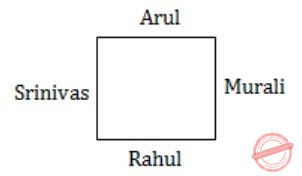Srinivas and Murali and Arul and Rahul are opposite to each other.
They face away from the center of tableEven in this case,
Srinivas and Murali and Arul and Rahul are opposite to each other.
 Question 59

Find the smallest number y such that y × 162 is a prefect cube.

 A 24 B 27 C 32 D 36
Aptitude       Numerical
Question 59 Explanation:
Prime factorize: 162
⇒ 162 = 2×3×3×3×3 = 33×(2×3)
For (2×3) to be a perfect cube, it should be multiplied by (22×32)
∴ Required number = y = 22×32 = 36
 Question 60

The probability that a k-digit number does NOT contain the digits 0, 5 or 9 is

 A 0.3k B 0.6k C 0.7k D 0.9k
Aptitude       Numerical
Question 60 Explanation:
Possibilities of doesn't contain 0,5,9 = (7)k
Total no.of possibilities = (10)k
Required probability = (7)k / (10)k = 0.7k
 Question 61

“The hold of the nationalist imagination on our colonial past is such that anything inadequately or improperly nationalist is just not history.”

Which of the following statements best reflects the author’s opinion?

 A Nationalists are highly imaginative. B History is viewed through the filter of nationalism. C Our colonial past never happened. D Nationalism has to be both adequately and properly imagined.
Aptitude       Verbal
Question 61 Explanation:
The author is explaining about the colonial past.
That represents the history of nationalism.
 Question 62

Six people are seated around a circular table. There are atleast two men and two women. There are at least three right-handed persons. Every woman has a left-handed person to her immediate right. None of the women are right-handed. The number of women at the table is

 A 2 B 3 C 4 D Cannot be determined
Aptitude       Numerical
Question 62 Explanation:
There are atleast two men and two women.
Atleast three right- handed persons.
None of the women are right-handed.
⇒ All the right-handed persons are men. Every woman has a left-handed person to her immediate right.
⇒ For this to happen, there must be three left-handed-persons and one of them should be a male.
As three persons are right-handed and those being men.
The number of women at the table = 2
 Question 63

The expression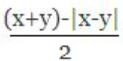is equal to

 A the maximum of x and y B the minimum of x and y C 1 D none of the above
Aptitude       Numerical
Question 63 Explanation:
As the given expression has modulus, let us consider:
Case-I: x > y
⇒ |x - y| = x - y
Expression ⇒ (x + y) - (x - y) / 2 = y
In this case value of expression is minimum of y.
Case-II: x < y
⇒ |x - y| = -(x - y)
Expression ⇒ (x + y) - (x - y) / 2
⇒ (x + y) - [-(x - y)]/2 ⇒ x
In this case value of expression is minimum of x.
Finally, the value of expression is minimum of x & y.
 Question 64

Arun, Gulab, Neel and Shweta must choose one shirt each from a pile of four shirts coloured red, pink, blue and white respectively. Arun dislikes the colour red and Shweta dislikes the colour white. Gulab and Neel like all the colours. In how many different ways can they choose the shirts so that no one has a shirt with a colour he or she likes?

 A 21 B 18 C 16 D 14
Aptitude       Numerical
Question 64 Explanation:
Total number of ways that each select one shirt without any condition = 4P4 = 4! = 24
Number of ways Arun chooses red or Shweta chooses white
= Number of ways Arun chooses red + Number of ways Shweta chooses white - Number of ways Arun chooses red and Shweta chooses white
= 3P3 + 3P3 - 2P2
= 3! + 3! - 2!
= 10
∴ Required number of ways = 24 - 10 = 14
 Question 65

A contour line joins locations having the same height above the mean sea level. The following is a contour plot of a geographical region. Contour lines are shown at 25 m intervals in this plot. If in a flood, the water level rises to 525 m, which of the villages P, Q, R, S, T get submerged?A P, Q B P, Q, T C R, S, T D Q, R, S
Aptitude       Numerical
Question 65 Explanation:
The contour lines are shown at 25m interval in this plot.
∴ From the plot,
P=575m
Q=525m
R=475m
S=425m
T=500m
For a village to be submerged, the water level should rise to level greater than the village.
If water level rises to 525m, villages which are at heights below 525m, get submerged.
Here, R, S, T get submerged.
There are 65 questions to complete.